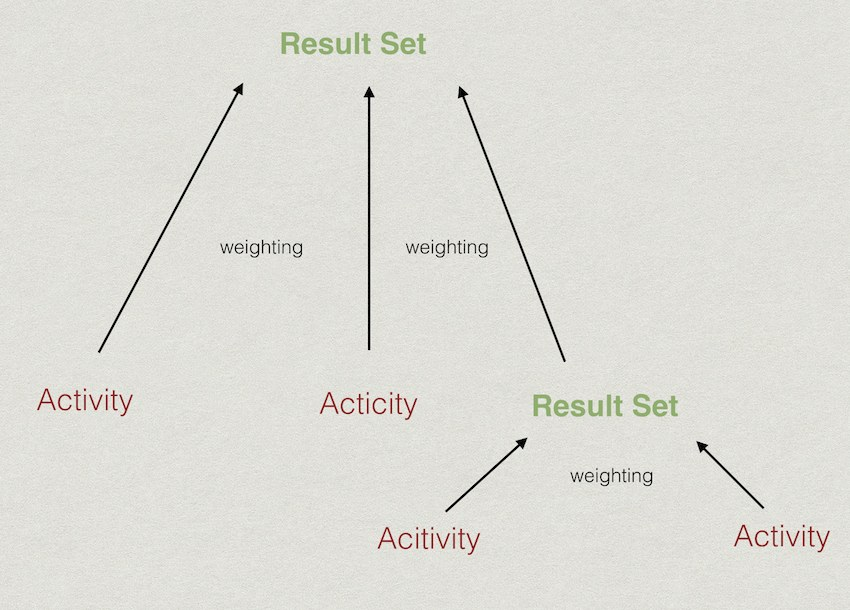# Setup – Markbook calculations explained

This article explains how to set up a markbook, and how marks are calculated.

To understand the basic concepts of the different types of results that are available, please read the creating results article.

### Mark Types

Activities, result sets and rule based results each have a mark type. There are two different mark types that a result can have:

#### Numeric Scale Type

A result with a numeric scale will allow a numeric mark to be captured into the markbook for that particular result. When a result has a numeric scale, a highest attainable mark (mark total) needs to be defined. For example, if a you would like to capture a mark for a test that had a mark total of 50 marks, you can create an Activity result, with a numeric scale and a highest attainable mark of 50. Only numerical values can then be entered into the markbook for that activity.

#### Custom Scale Type

Assess allows for the creation of custom scales. By default, the SA national codes and descriptors scale (1 – 7) is already loaded into Staffroom.

When a result has a scale mark type, a further option allows you to select which scale to use for that result. The markbook now only allows you to enter marks within that scale for that particular result. A custom scale can consist of numerical values, like the 1 – 7 scale, but can also have alphabetical values, like A – F.

### Mark Calculations

Markbooks can be set up to automatically to calculate results that have been achieved. Results can be grouped together to calculate towards another result using Result Sets. A result set can consist of any combination of other results – so activities and other result sets can be grouped together.#### Calculation Type

In the illustration above, you can see that results can be grouped together in any way, and each result set’s mark is calculated using a weighting between the different results that lead up to it. This weighting is defined by the “calculation type” setting.

The different calculation types are:

• Average: Each result in the result set counts equally towards the result set’s mark, by calculating an average mark.
• Manual Weighting: If the manual calculation type is selected, you can define the weighting for each individual result in the result set. This weighting is a ratio between the different results. So for example, a result set can have three results in it, with a weighting ratio of: 10 : 20 : 30. In this example the second result counts twice as much, and the third result three times as much towards the result set mark. (NB: You do not have to force this to be a percentage)
• Weighted Mean: The mark totals for each individual result in the result set are used as the weightings. For example, if a result set consists of results that are marked out of 15, 35 and 50 respectively, then the weightings of those results are 15 : 35 : 50.
• Predominant Value (Mode): This will choose the mark that occurs the most in a set of results.
• Median: This will choose the mark that is in the middle of a set of results, when those marks are ordered.
• Range: This will choose the difference between the highest and the lowest mark achieved in the set of results.
• Raw Sum: The raw sum calculation adds up all the raw marks that have been achieved for all the results in the set. The result set itself takes on a mark total of the sum of all it’s child results’ mark totals. For example, the result set consists of results with totals of 10, 25 and 50. The result set’s mark total will be 85. The mark that a student achieved for that result set would be the raw marks added up. So if they achieved 8, 20 and 45 respectively for those results, then their mark would be 73/85.
• Highest: This chooses the highest mark achieved in the set of results.
• Lowest: This chooses the lowest mark achieved in the set of results.
• Sum: This works differently to the raw sum in that the result set’s mark total doesn’t consist of the child results’ mark totals. The normal sum, adds up each mark achieved individually, and calculates the result set according to what it’s highest attainable mark is set at.

### Result Sets and different Mark Types

As each result can have it’s own mark type, it would raise the question whether one could combine results with different mark types, or convert between different mark types. The short answer is, yes!

Due to the fact that each scale level (mark) in a custom scale is linked to a percentage range, Staffroom knows how to convert those to numerical values and vice versa. So if a result set consists of results with different mark types, the resulting mark will be converted to the mark type that the result set itself is in.

For example:

A result set might have a scale mark type, and use the SA national codes and descriptors scale (ie. a mark from 1 – 7 with each of those marks falling within a range of percentages).

This result set might have three activities all with a numeric mark type, and marks out of 15, 25 and 55 respectively. If the calculation type is average, staffroom calculates an average of the three marks that have been achieved, and converts the calculated average to a mark in the 1 – 7 scale.